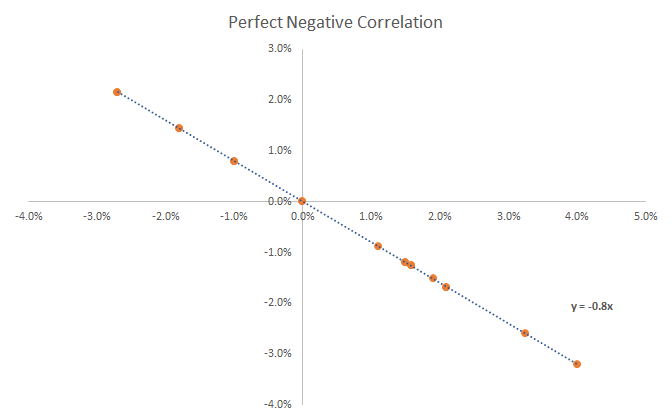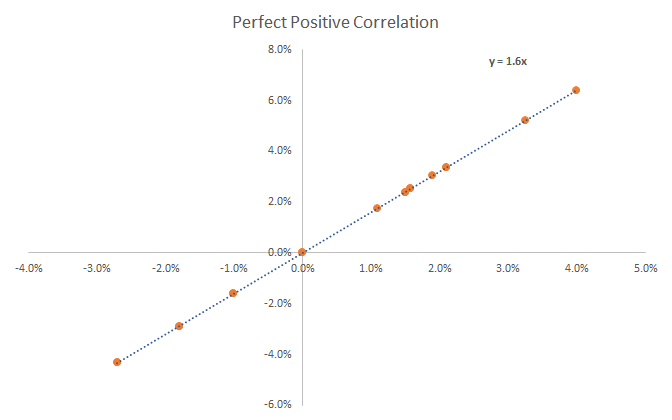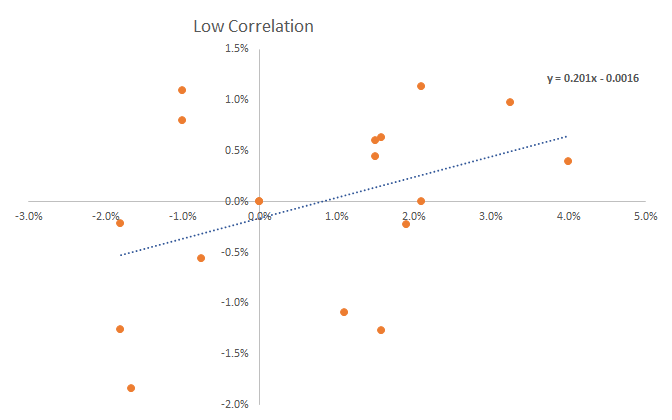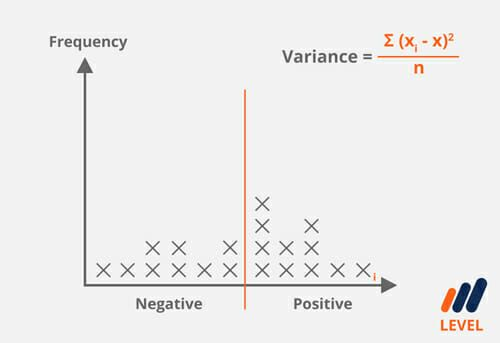# Negative Correlation

One variable rises, another falls

## What is a Negative Correlation?

A negative correlation is a relationship between two variables that move in opposite directions. In other words, when variable A increases, variable B decreases. A negative correlation is also known as an inverse correlation.

Two variables can have varying strengths of negative correlation. The variable A could be strongly negatively correlated with B, and may have a correlation coefficient of -0.9. This means that for every change in unit of variable B, variable A experiences a decrease by 0.9. As another example, these variables could also have a weak negative correlation. A coefficient of -0.2 means that for every unit change in variable B, variable A experiences a decrease, but only slightly, by 0.2.

### Negative, Positive, and Low Correlation Examples

Let’s start with a graph of perfect negative correlation.  As you can see in the graph below, the equation of the line is y = -0.8x.  What that means is if Stock Y is up 1.0%, stock X will be down 0.8%.  This relationship is perfectly inverse as they always move in opposite directions.  Learn more about this in CFI’s online financial math course.Now let’s look at a graph with perfect positive correlation.  In the graph below you can that if Stock Y is up 1.0%, Stock X is up 1.6%, and since they move in the same directory every time.  Learn about correlations in CFI’s online financial math course.Finally, let’s look at another example, this time of two low correlated assets.  As you can see, the dots that are very dispersed and none of them lie on the line of best fit.  For these two stocks, there is almost no correlation between the return of Stock Y and the return of Stock X. The two securities move completely independent of one another.Correlation is covered in more detail in CFI’s math for finance professionals

### What are the benefits of negatively correlated assets in portfolios?

The concept of negative correlation is important for investors or analysts who are considering adding new investments to their portfolio. When market uncertainty is high, a common consideration is re-balancing portfolios by replacing some securities that have position correlation with those that have a negative correlation.

The portfolio movements offset each other, reducing risk but also return. After the market uncertainty has diminished, investors can start closing offset positions. An example of negatively correlated securities would be a stock and put option on the stock , which gains in value as the stock’s price falls.

### Negative Coefficient

A pair of instruments will always have a coefficient that lies between -1 to 1. A coefficient below zero indicates a negative correlation. When two instruments have a correlation of -1, these instruments have a perfectly inverse relationship. If instrument A moves up by \$1, instrument B will move down by \$1.

In another example, if the correlation between the EUR/USD exchange rate and USD/CHF exchange rate has a coefficient of -0.85, for every 100 points the EUR/USD moves up, the USD/CHF will move down by 85.### Examples of negative correlation assets

Here are some common examples of negatively correlated relationships between assets:

• Oil prices and airline stocks
• Gold prices and stock markets (most of the time, but not always)
• Any type of insurance payoff

Thank you for reading CFI’s guide to inversely correlated assets in investing and finance.  To keep learning more, CFI highly recommends:

• Risk and return
• Credit default swaps
• Efficient market hypothesis
• Financial modeling guide

### Financial Analyst Training

Get world-class financial training with CFI’s online certified financial analyst training program!

Gain the confidence you need to move up the ladder in a high powered corporate finance career path.

Learn financial modeling and valuation in Excel the easy way, with step-by-step training.